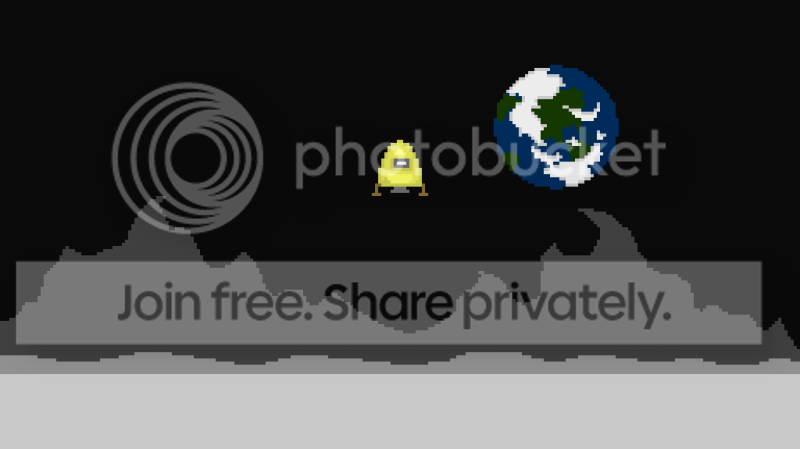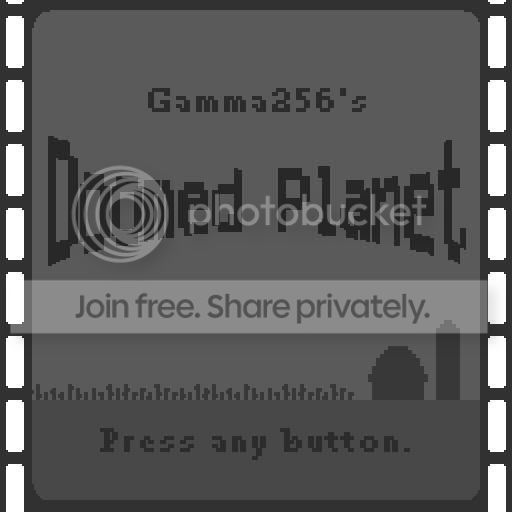-=+=- -=+=- -=+=- -=+=- -=+=- -=+=- -=+=- -=+=- -=+=- -=+=- -=+=- -=+=- -=+=- -=+=- -=+=- -=+=- -=+=- -=+=- -=+=- -=+=- -=+=- -=+=- -=+=- -=+=- -=+=- -=+=- -=+=- -=+=- -=+=- -=+=- (c) WidthPadding Industries 1987 0|309|0 -=+=- -=+=- -=+=- -=+=- -=+=- -=+=- -=+=- -=+=- -=+=- -=+=- -=+=- -=+=- -=+=- -=+=- -=+=- -=+=- -=+=- -=+=- -=+=- -=+=- -=+=- -=+=- -=+=- -=+=- -=+=- -=+=- -=+=- -=+=- -=+=- -=+=-

blanko1324# To The Moon!

18th February 2010A Game for Gamma 4

blanko1324# Spaceship Sim

14th January 2010A small space project I've been fooling around with. (Inspired by Star Trek)

blanko1324# Shambo

16th June 2008A Game of Super Strategy Maybe. (VER 0.7)

blanko1324# Doomed Planet!

28th October 2007...has NOT been rejected!

blanko1324# Detonate 3

24th December 2006Updated 1/21/07

More - Older Posts